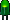• Home• Patreon• Links• Books
Number Converting:

# 4. Octal Number Conversion

(article continued from previous page)

## Octal to Binary

Converting from octal to binary is as easy as converting from binary to octal. Simply look up each octal digit to obtain the equivalent group of three binary digits.

 Octal: 0 1 2 3 4 5 6 7 Binary: 000 001 010 011 100 101 110 111

 Octal  = 3 4 5 Binary = 011 100 101 = 011100101 binary

When converting from octal to hexadecimal, it is often easier to first convert the octal number into binary and then from binary into hexadecimal. For example, to convert 345 octal into hex:

(from the previous example)

 Octal  = 3 4 5 Binary = 011 100 101 = 011100101 binary

Drop any leading zeros or pad with leading zeros to get groups of four binary digits (bits):
Binary 011100101 = 1110 0101

Then, look up the groups in a table to convert to hexadecimal digits.

 Binary: 0000 0001 0010 0011 0100 0101 0110 0111 Hexadecimal: 0 1 2 3 4 5 6 7
 Binary: 1000 1001 1010 1011 1100 1101 1110 1111 Hexadecimal: 8 9 A B C D E F

 Binary = 1110 0101 Hexadecimal = E 5 = E5 hex

Therefore, through a two-step conversion process, octal 345 equals binary 011100101 equals hexadecimal E5.

## Octal to Decimal

Converting octal to decimal can be done with repeated division.

1. Start the decimal result at 0.
2. Remove the most significant octal digit (leftmost) and add it to the result.
3. If all octal digits have been removed, you’re done. Stop.
4. Otherwise, multiply the result by 8.
5. Go to step 2.
 Octal Digits Operation Decimal Result Operation Decimal Result 345 +3 3 × 8 24 45 +4 28 × 8 224 5 +5 229 done.

The conversion can also be performed in the conventional mathematical way, by showing each digit place as an increasing power of 8.

345 octal = (3 * 82) + (4 * 81) + (5 * 80) = (3 * 64) + (4 * 8) + (5 * 1) = 229 decimal